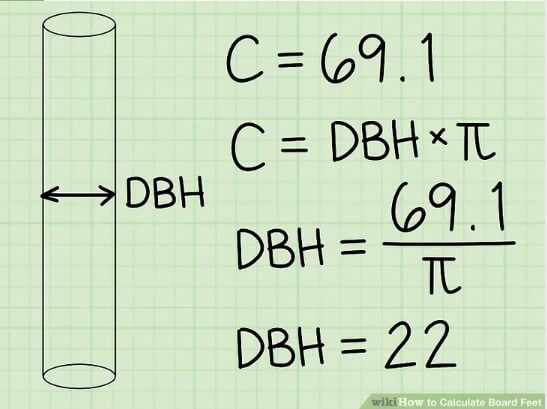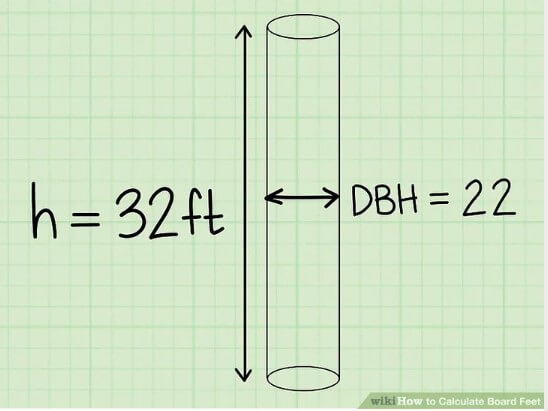# Board Foot Calculator

## What is Board foot (FBM)?

Board foot or board-foot is the unit measurement of lumber to calculate the volume of lumber. This is basically a unit for the lumber i.e. one-foot in length, one foot wide and one inch thick.

Board foot is also shortened as FBM (footboard measure), BF and BDFT.

One board foot equal to:

• 1 ft × 1 ft × 1 in
• 144 in3
• 1/12 ft3
• 12 in × 12 in × 1 in
• 30.48 cm × 30.48 cm × 2.54 cm
• ≈ 2.36 liters
• ≈ 0.00236 cubic meters
• ≈ 2360 cm3

## How to figure Board Feet?

There are two ways to Calculate board feet:

1. Manually by using formulas
2. Using the online board feet calculator

The manual calculation is of two types; measurement of a tree or log and the calculation of FBM of supplied boards.

Here are easy steps to calculate board feet of a tree:

### Step 1: find the diameter

Find the diameter of the log by the use of a flexible measuring tape, tree caliper or tree diameter tape. measure precisely by using a string, wrapping it with the tree and cut it where it meets or use a Biltmore scale for DBH (diameter at breast height) measure.Image Source: WikiHow

### Step 2: Find the height or length of the log in feet

Use your measuring instrument to find the height. Tree length is measured in 16-foot to measure board feet. Log height/length is typically measured in even number like 2-foot, 4-foot, 6-foot, 8-foot increments and so on.

.Image Source: WikiHow

### Step 3: Use the Doyle scale

Doyle scale is used to calculate board feet of log and tree. Simply embed your estimations into the Doyle scale. Insert the diameter at breast height (DBH) and various number of 16-foots logs in the tree or use the diameter measured at the small end of the log inside the bark and use it to ascertain the total volume of the board.

## Example

• Let say DBH is 22 and height 32 feet or there are two 16-foot logs

DBH= 22

Height= 32 ÷ 16 =2

Using the Doyle scale you will find out the board feet is = 295 board feet

To find the board feet of boards the formulas and method are different here is how you can calculate:

### Step 1: Use the rough size

Prefer using the rough size of the boards instead of the actual size. The insignificant, size of a board states to the dimensions the maker will supply you with, e.g. “3 x 5” which means 3 inches by 5 inches. furthermore, after the remaining process, the board’s actual measurements are closer to 2.5 inches by 4.5 inches.

Using the rough size of boards will give precise results as compared to the actual size so using rough size is more accurate.

### Step 2: measure thickness and length

Measure width and thickness in inches and the length of the board in feet. Use a measuring tape to find thickness and width and then measure the length in feet rather than inches. These typical measures are used for lumber calculations only.

### Step 3: multiply and divide

After measuring thickness and width and length, multiply the thickness with width and length and divide the result with 12.

Board Feet = \dfrac{L \times W \times T }{12}12L× W×T
After measuring Board Foot you can calculate the total board cost as well.

Total cost= Total BF × cost of one BF

Another Example

If you have 10 boards of the same size each is 2 inches by 8 inches by 6 feet. Multiply them i.e. 2 into 8 into 6 equals 96 and dividing it by 12 equals 8 and this is the board feet of a single board and after that you can multiply it with a total number of boards to find the total board feet.

## Calculate Board Feet Using Online Calculator:

Calculating board feet was never easy until our developer made this most precise online lumber calculator (also known as Board ft. calculator) to easily calculate the board feet and total board feet with the total cost of the boards.

All you have to do is just know the dimensions of the board, number of boards and cost you want to calculate and insert the reading inside the provided area and click on the “calculate” button and there you go with the answers in seconds. You will be provided with the total cost and total board feet.

Using our tool is free of cost and can be used multiple times as per person demand.

#### Need Custom Calculator?

With Over Online Tools, eCalculator.co Helping Millions of Students, Teachers, Businessmen & Nutritionists Every Month.